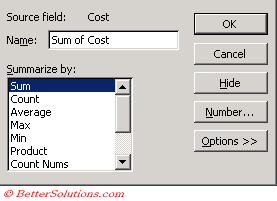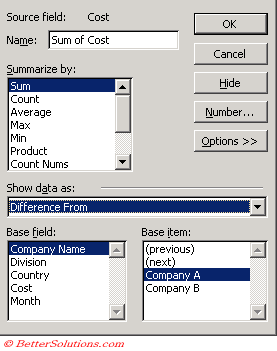# Summary Functions

### Field Calculations

If the data area contains numerical values then the SUM() function is used by default
If the data area contains non numerical values then the COUNT() function is used.
What are the options ?
You can specify which fields to include and the type of calculations used on those fields.

For each combination of values in the row and column fields, the data field takes on a different value and this value appears in the data area.

### Default Calculations

It is possible to use other function in order to summarise your data.
There are actually a choice of eleven different aggregate functions that can be used in your pivot table.SUM This is the default function used when the data area contains numeric values. The total value of the numbers in a list or cell range. COUNT This is the default function used when the data area contains non numeric values. The number of numeric values in a list or array of numbers. COUNTA Count Nums The number of non blank cells in a list or cell range. AVERAGE The arithmetic mean of a list or array of numbers. MAX The largest value in a list or array of numbers. MIN The smallest value in a list or array of numbers. PRODUCT The product of all the numbers in a list or cell range. STDDEV The standard deviation based on a sample. STDDEVP The standard deviation based on an entire population. VAR The compound variance based on a sample. VARP The variance based on an entire population.

When you change the function, the Data area will reflect the changes automatically.
It is possible to customise the selected function by adding some calculation options on the pivot table field dialog box.
In addition to the eleven functions that are provide by default you can also create your own custom calculations.

### Custom Calculations

There are also a large number of custom calculations which you can use including running totals and item percentages.

Some of these calculations require a field to use as well as the value for the field.
To apply a custom calculation (PivotTable > Field Settings) "Options".When you select a member of the Base Field, the corresponding items will automatically be displayed in the Base Item.

 Normal Default Difference From Calculates the difference between two cells. % of Calculates the percentage of a cell to a selected base value. % Difference From Calculates the difference between two cell values. Running Total in Calculates and displays the running total in each cell. % of row Calculates the percentage of the cell value to the total row. % of column Calculates the percentage of the cell value to the total column. % of total Calculates the percentage of the value of the grand total. Index Calculates the index value of the cell value.

### Important

You also have the option of creating your own calculated fields and items.